## Momentum and Collisions Review

### Part A: Multiple-Multiple Choice

1. Which of the following statements are true about momentum?

1. Momentum is a vector quantity.
2. The standard unit on momentum is the Joule.
3. An object with mass will have momentum.
4. An object which is moving at a constant speed has momentum.
5. An object can be traveling eastward and slowing down; its momentum is westward.
6. Momentum is a conserved quantity; the momentum of an object is never changed.
7. The momentum of an object varies directly with the speed of the object.
8. Two objects of different mass are moving at the same speed; the more massive object will have the greatest momentum.
9. A less massive object can never have more momentum than a more massive object.
10. Two identical objects are moving in opposite directions at the same speed. The forward moving object will have the greatest momentum.
11. An object with a changing speed will have a changing momentum.

2. Which of the following are true about the relationship between momentum end energy?

1. Momentum is a form of energy.
2. If an object has momentum, then it must also have mechanical energy.
3. If an object does not have momentum, then it definitely does not have mechanical energy either.
4. Object A has more momentum than object B. Therefore, object A will also have more kinetic energy.
5. Two objects of varying mass have the same momentum. The least massive of the two objects will have the greatest kinetic energy.

3. Which of the following statements are true about impulse?

1. Impulse is a force.
2. Impulse is a vector quantity.
3. An object which is traveling east would experience a westward directed impulse in a collision.
4. Objects involved in collisions encounter impulses.
5. The Newton is the unit for impulse.
6. The kg•m/s is equivalent to the units on impulse.
7. An object which experiences a net impulse will definitely experience a momentum change.
8. In a collision, the net impulse experienced by an object is equal to its momentum change.
9. A force of 100 N acting for 0.1 seconds would provide an equivalent impulse as a force of 5 N acting for 2.0 seconds.

4. Which of the following statements are true about collisions?

1. Two colliding objects will exert equal forces upon each other even if their mass is significantly different.
2. During a collision, an object always encounters an impulse and a change in momentum.
3. During a collision, the impulse which an object experiences is equal to its velocity change.
4. The velocity change of two respective objects involved in a collision will always be equal.
5. While individual objects may change their velocity during a collision, the overall or total velocity of the colliding objects is conserved.
6. In a collision, the two colliding objects could have different acceleration values.
7. In a collision between two objects of identical mass, the acceleration values could be different.
8. Total momentum is always conserved between any two objects involved in a collision.
9. When a moving object collides with a stationary object of identical mass, the stationary object encounters the greater collision force.
10. When a moving object collides with a stationary object of identical mass, the stationary object encounters the greater momentum change.
11. A moving object collides with a stationary object; the stationary object has significantly less mass. The stationary object encounters the greater collision force.
12. A moving object collides with a stationary object; the stationary object has significantly less mass. The stationary object encounters the greater momentum change.

5. Which of the following statements are true about elastic and inelastic collisions?

1. Perfectly elastic and perfectly inelastic collisions are the two opposite extremes along a continuum; where a particular collision lies along the continuum is dependent upon the amount kinetic energy which is conserved by the two objects.
2. Most collisions tend to be partially to completely elastic.
3. Momentum is conserved in an elastic collision but not in an inelastic collision.
4. The kinetic energy of an object remains constant during an elastic collision.
5. Elastic collisions occur when the collision force is a non-contact force.
6. Most collisions are not inelastic because the collision forces cause energy of motion to be transformed into sound, light and thermal energy (to name a few).
7. A ball is dropped from rest and collides with the ground. The higher that the ball rises upon collision with the ground, the more elastic that the collision is.
8. A moving air track glider collides with a second stationary glider of identical mass. The first glider loses all of its kinetic energy during the collision as the second glider is set in motion with the same original speed as the first glider. Since the first glider lost all of its kinetic energy, this is a perfectly inelastic collision.
9. The collision between a tennis ball and a tennis racket tends to be more elastic in nature than a collision between a halfback and linebacker in football.

### Part B: Multiple Choice

6. Which of the following objects have momentum? Include all that apply.

a. An electron is orbiting the nucleus of an atom.

b. A UPS truck is stopped in front of the school building.

c. A Yugo (a compact car) is moving with a constant speed.

d. A small flea walking with constant speed across Fido's back.

e. The high school building rests in the middle of town.

7. A truck driving along a highway road has a large quantity of momentum. If it moves at the same speed but has twice as much mass, its momentum is ________________.

 a. zero b. quadrupled c. doubled d. unchanged

8. TRUE or FALSE:

A ball is dropped from the same height upon various flat surfaces. For the same collision time, impulses are smaller when the most bouncing take place.
 a. True b. False

9. Consider a karate expert. During a talent show, she executes a swift blow to a cement block and breaks it with her bare hand. During the collision between her hand and the block, the ___.

1. time of impact on both the block and the expert's hand is the same
2. force on both the block and the expert's hand have the same magnitude
3. impulse on both the block and the expert's hand have the same magnitude
4. all of the above.
5. none of the above.

10. It is NOT possible for a rocket to accelerate in outer space because ____. List all that apply.

1. there is no air in space
2. there is no friction in space
3. there is no gravity in outer space
4. ... nonsense! Rockets do accelerate in outer space.

11. In order to catch a ball, a baseball player naturally moves his or her hand backward in the direction of the ball's motion once the ball contacts the hand. This habit causes the force of impact on the players hand to be reduced in size principally because ___.

1. the resulting impact velocity is lessened
2. the momentum change is decreased
3. the time of impact is increased
4. the time of impact is decreased
5. none of these

12. Suppose that Paul D. Trigger fires a bullet from a gun. The speed of the bullet leaving the muzzle will be the same as the speed of the recoiling gun ____.

1. because momentum is conserved
2. because velocity is conserved
3. because both velocity and momentum are conserved
4. only if the mass of the bullet equals the mass of the gun
5. none of these

13. Suppose that you're driving down the highway and a moth crashes into the windshield of your car. Which undergoes the greater change is momentum?

 a. the moth b. your car c. both the same

14. Suppose that you're driving down the highway and a moth crashes into the windshield of your car. Which undergoes the greater force?

 a. the moth b. your car c. both the same

15. Suppose that you're driving down the highway and a moth crashes into the windshield of your car. Which undergoes the greater impulse?

 a. the moth b. your car c. both the same

16. Suppose that you're driving down the highway and a moth crashes into the windshield of your car. Which undergoes the greater acceleration?

 a. the moth b. your car c. both the same

17. Three boxes, X, Y, and Z, are at rest on a table as shown in the diagram at the right. The weight of each box is indicated in the diagram. The net or unbalanced force acting on box Y is _____.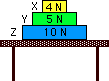a. 4 N down b. 5 N down c. 5 N up d. 10 N up e. zero

18. In a physics experiment, two equal-mass carts roll towards each other on a level, low-friction track. One cart rolls rightward at 2 m/s and the other cart rolls leftward at 1 m/s. After the carts collide, they couple (attach together) and roll together with a speed of _____________. Ignore resistive forces.

 a. 0.5 m/s b. 0.33 m/s c. 0.67 m/s d. 1.0 m/s e. none of these

19. A physics cart rolls along a low-friction track with considerable momentum. If it rolls at the same speed but has twice as much mass, its momentum is ____.

 a. zero b. four times as large c. twice as large d. unchanged

20. The firing of a bullet by a rifle causes the rifle to recoil backwards. The speed of the rifle's recoil is smaller than the bullet's forward speed because the ___.

 a. force against the rifle is relatively small b. speed is mainly concentrated in the bullet c. rifle has lots of mass d. momentum of the rifle is unchanged e. none of these

21. Two objects, A and B, have the same size and shape. Object A is twice as massive as B. The objects are simultaneously dropped from a high window on a tall building. (Neglect the effect air resistance.) The objects will reach the ground at the same time but object A will have a greater ___. Choose all that apply.

 a. speed b. acceleration c. momentum d. none of the above quantities will be greater

22. Cars are equipped with padded dashboards. In collisions, the padded dashboards would be safer than non-padded ones because they ____. List all that apply.

 a. increase the impact time b. decrease an occupant's impulse c. decrease the impact force d. none of the above

23. A 4 kg object has a momentum of 12 kg•m/s. The object's speed is ___ m/s.

 a. 3 b. 4 c. 12 d. 48 e. none of these.

24. A wad of chewed bubble gum is moving with 1 unit of momentum when it collides with a heavy box that is initially at rest. The gum sticks to the box and both are set in motion with a combined momentum that is ___.

 a. less than 1 unit b. 1 unit c. more than 1 unit d. not enough information

25. A relatively large force acting for a relatively long amount of time on a relatively small mass will produce a relatively ______. List all that apply.

 a. small velocity change b. large velocity change c. small momentum change d. small acceleration

26. Consider the concepts of work and energy (presuming you have already studied it) and those of impuse and momentum. Force and time is related to momentum change in the same manner as force and displacement pertains to ___________.

 a. impulse b. work c. energy change d. velocity e. none of these.

27. A 5-N force is applied to a 3-kg ball to change its velocity from +9 m/s to +3 m/s. This impulse causes the momentum change of the ball to be ____ kg•m/s.

 a. -2.5 b. -10 c. -18 d. -45 e. none of these

28. A 5-N force is applied to a 3-kg ball to change its velocity from +9 m/s to +3 m/s. The impulse experienced by the ball is ____ N•s.

 a. -2.5 b. -10 c. -18 d. -45 e. none of these

29. A 5-N force is applied to a 3-kg ball to change its velocity from +9 m/s to +3 m/s. The impulse is encountered by the ball for a time of ____ seconds.

 a. 1.8 b. 2.5 c. 3.6 d. 10 e. none of these

30. When a mass M experiences a velocity change of v in a time of t, it experiences a force of F. Assuming the same velocity change of v, the force experienced by a mass of 2M in a time of (1/2)t is ____.

 a. 2F b. 4F c. (1/2)*F d. (1/4)*F e. none of these

31. When a mass M experiences a velocity change of v in a time of t, it experiences a force of F. Assuming the same velocity change of v, the force experienced by a mass of 2M in a time of (1/4)t is ____.

 a. 2F b. 8F c. (1/2)*F d. (1/8)*F e. none of these

32. When a mass M experiences a velocity change of v in a time of t, it experiences a force of F. Assuming the same velocity change of v, the force experienced by a mass of (1/2)M in a time of (1/2)t is ____.

 a. 2F b. 4F c. (1/2)*F d. (1/4)*F e. none of these

33. When a mass M experiences a velocity change of v in a time of t, it experiences a force of F. Assuming the same velocity change of v, the force experienced by a mass of (1/2)M in a time of 4t is ____.

 a. 2F b. 8F c. (1/2)*F d. (1/8)*F e. none of these

34. A 0.5-kg ball moving at 5 m/s strikes a wall and rebounds in the opposite direction with a speed of 2 m/s. If the impulse occurs for a time duration of 0.01 s, then the average force (magnitude only) acting upon the ball is ____ Newtons.

 a. 0.14 b. 150 c. 350 d. 500 e. none of these

35. If mass and collision time are equal, then impulses are greater on objects which rebound (or bounce).

 a. TRUE b. FALSE

36. Consider the head-on collision between a lady bug and the windshield of a high speed bus. Which of the following statements are true? List all that apply.

1. The magnitude of the force encountered by the bug is greater than that of the bus.
2. The magnitude of the impulse encountered by the bug is greater than that of the bus.
3. The magnitude of the momentum change encountered by the bug is greater than that of the bus.
4. The magnitude of the velocity change encountered by the bug is greater than that of the bus.
5. The magnitude of the acceleration encountered by the bug is greater than that of the bus.

### Part C: Diagramming and Analysis

For Questions #37-#40: Consider the before- and after-collision momentum vectors in the diagram below. Determine the magnitude and direction of the system momentum before and after the collision and identify whether or not momentum is conserved. Finally, determine the magnitude and direction of the net external impulse encountered by the system during the collision.

 37.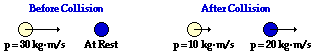System Momentum Before Collision: _________________________ System Momentum After Collision: _________________________ Is momentum conserved? _________________________ Net External Impulse During Collision: _________________________

 38.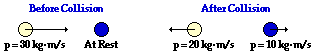System Momentum Before Collision: _________________________ System Momentum After Collision: _________________________ Is momentum conserved? _________________________ Net External Impulse During Collision: _________________________

 39.System Momentum Before Collision: _________________________ System Momentum After Collision: _________________________ Is momentum conserved? _________________________ Net External Impulse During Collision: _________________________

 40.System Momentum Before Collision: _________________________ System Momentum After Collision: _________________________ Is momentum conserved? _________________________ Net External Impulse During Collision: _________________________

For Questions #41-#44: Repeat the procedure performed in questions #37-40. Note that these diagrams give velocity and mass values before and after the collision.

 41.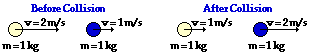System Momentum Before Collision: _________________________ System Momentum After Collision: _________________________ Is momentum conserved? _________________________ Net External Impulse During Collision: _________________________

 42.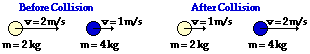System Momentum Before Collision: _________________________ System Momentum After Collision: _________________________ Is momentum conserved? _________________________ Net External Impulse During Collision: _________________________

 43.System Momentum Before Collision: _________________________ System Momentum After Collision: _________________________ Is momentum conserved? _________________________ Net External Impulse During Collision: _________________________

 44.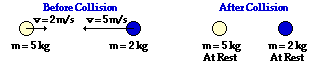System Momentum Before Collision: _________________________ System Momentum After Collision: _________________________ Is momentum conserved? _________________________ Net External Impulse During Collision: _________________________

For Questions #45-#49, determine the unknown velocity value. Assume that the collisions occur in an isolated system.

 45.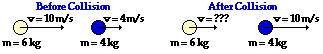46.47.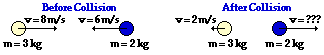48.49.For Questions #50-#52, determine the total kinetic energy of the system before and after the collision and identify the collision as being either perfectly elastic, partially inelastic/elastic or perfectly inelastic.

 50.Total System Kinetic Energy Before Collision: ______________ Total System Kinetic Energy After Collision: ______________ Perfectly Elastic, Partially Inelastic/Elastic or Perfectly Inelastic? ______________

 51.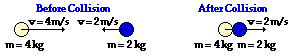Total System Kinetic Energy Before Collision: ______________ Total System Kinetic Energy After Collision: ______________ Perfectly Elastic, Partially Inelastic/Elastic or Perfectly Inelastic? ______________

 52.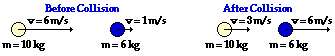Total System Kinetic Energy Before Collision: ______________ Total System Kinetic Energy After Collision: ______________ Perfectly Elastic, Partially Inelastic/Elastic or Perfectly Inelastic? ______________

### Part D: Qualitative Relationships Between Variables

53. An object with a mass M and a velocity v has a momentum of 32 kg•m/s. An object with a mass of ...

1. ... 2M and a velocity of 2v would have a momentum of ____ kg•m/s.
2. ... 2M and a velocity of 0.5v would have a momentum of ____ kg•m/s.
3. ... 0.5M and a velocity of 2v would have a momentum of ____ kg•m/s.
4. ... 0.5M and a velocity of 0.5v would have a momentum of ____ kg•m/s.
5. ... 4M and a velocity of v would have a momentum of ____ kg•m/s.
6. ... 4M and a velocity of 0.5v would have a momentum of ____ kg•m/s.
7. ... 0.5M and a velocity of 4v would have a momentum of ____ kg•m/s.
8. ... 3M and a velocity of 2v would have a momentum of ____ kg•m/s.

54. An object with a mass M and a velocity v undergoes a collision and encounters a force of F for a time of t. The collision brings the object to a final rest position ...

1. ... If the object had a mass of 2M and a velocity of v, then it would need an impulse which is ____ F•t in order to be brought to rest. (Place a multiplying factor in the blank.)
2. ... If the same object encountered a force of 2F, then it would bring it to rest in a time of ____ t, The impulse would be _____ (twice the, one-half the, the same) size and the momentum change would be _____ (twice the, one-half the, the same) size.
3. ... If the same object encountered a force of 10F, then it would bring it to rest in a time of ____ t, The impulse would be _____ (ten times the, one-tenth the, the same) size and the momentum change would be _____ (ten times the, one-tenth the, the same) size.
4. ... If the same object encountered a force of 0.2F, then it would bring it to rest in a time of ____ t, The impulse would be _____ (five times the, one-fifth the, the same) size and the momentum change would be _____ (five times the, one-fifth the, the same) size.
5. ... If the object had a mass of 2M and a velocity of v encountered a force of 4F, then it would be brought to rest in a time of ____ t. The impulse would be _____ times the original impulse (put a multiplier in the blank) and the momentum change would be _____ times the original impulse (put a multiplier in the blank).
6. ... If the object had a mass of 2M and a velocity of 2v encountered a force of 4F, then it would be brought to rest in a time of ____ t. The impulse would be _____ times the original impulse (put a multiplier in the blank) and the momentum change would be _____ times the original impulse (put a multiplier in the blank).
7. ... If the object had a mass of 0.5M and a velocity of 4v encountered a force of 2F, then it would be brought to rest in a time of ____ t. The impulse would be _____ times the original impulse (put a multiplier in the blank) and the momentum change would be _____ times the original impulse (put a multiplier in the blank).

55. Two carts are placed next to each other on a low-friction track. The carts are equipped with a spring-loaded mechanism which allows them to impart an impulse to each other. Cart A has a mass of M and Cart B has a mass of M. The spring-loaded mechanism is engaged and then released. The impulse causes Cart A to be propelled forward with a velocity of 40 cm/s.

1. Cart B will be propelled backward with a velocity of ___ cm/s.
2. ... If Cart B had a mass of 2M then it would be propelled backwards with a velocity of ___ cm/s.
3. ... If Cart B had a mass of 0.5M then it would be propelled backwards with a velocity of ___ cm/s.
4. ... If Cart B has a mass of 2M then it would be propelled backwards with a momentum which is ____ (two times the, one-half the, the same as the) original momentum.
5. ... If Cart B has a mass of 2M then it would encounter an impulse which is ____ (two times the, one-half the, the same as the) original impulse.

56. A cart with a mass of M is moving along a low-friction track with a speed of 60 cm/s. A brick is gently dropped from rest upon the cart. After the collision the cart and brick move together.

1. ... If the brick has a mass of 2M, then the post-collision speed of the two objects will be ____ cm/s.
2. ... If the brick has a mass of 3M, then the post-collision speed of the two objects will be ____ cm/s.
3. ... If the brick has a mass of 4M, then the post-collision speed of the two objects will be ____ cm/s.
4. ... If the brick has a mass of 5M, then the post-collision speed of the two objects will be ____ cm/s.
5. ... If the brick has a mass of 0.5M, then the post-collision speed of the two objects will be ____ cm/s.
6. ... If the brick has a mass of 0.25M, then the post-collision speed of the two objects will be ____ cm/s.

### Part E: Problem-Solving

57. A 0.530-kg basketball hits a wall head-on with a forward speed of 18.0 m/s. It rebounds with a speed of 13.5 m/s. The contact time is 0.100 seconds. (a) determine the impulse with the wall, (b) determine the force of the wall on the ball.

58. A 4.0-kg object has a forward momentum of 20. kg•m/s. A 60. N•s impulse acts upon it in the direction of motion for 5.0 seconds. A resistive force of 6.0 N then impedes its motion for 8.0 seconds. Determine the final velocity of the object.

59. A 3.0-kg object is moving forward with a speed of 6.0 m/s. The object then encounters a force of 2.5 N for 8.0 seconds in the direction of its motion. The object then collides head-on with a wall and heads in the opposite direction with a speed of 5.0 m/s. Determine the impulse delivered by the wall to the object.

60. A 46-gram tennis ball is launched from a 1.35-kg homemade cannon. If the cannon recoils with a speed of 2.1 m/s, determine the muzzle speed of the tennis ball.

61. A 2.0-kg box is attached by a string to a 5.0-kg box. A compressed spring is placed between them. The two boxes are initially at rest on a friction-free track. The string is cut and the spring applies an impulse to both boxes, setting them in motion. The 2.0-kg box is propelled backwards and moves 1.2 meters to the end of the track in 0.50 seconds. Determine the time it takes the 5.0-kg box to move 0.90 meters to the opposite end of the track.

62. Two children are playing with a large snowball while on ice skates on a frozen pond. The 33-kg child tosses the 5.0-kg snowball, imparting a horizontal speed of 5.0 m/s to it. The 33-kg child is 4.0 meters from a 28-kg child and 8.0 meters from the edge of the pond (located behind him). Assuming negligible friction, how much time elapses between when the 28-kg child gets hit by the snowball and when the 33-kg child reaches the edge of the pond?

63. A 2.8-kg physics cart is moving forward with a speed of 45 cm/s. A 1.9-kg brick is dropped from rest and lands on the cart. The cart and brick move together across the horizontal surface. Assume an isolated system.

a. Determine the post-collision speed of the cart and the brick.

b. Determine the momentum change of the cart.

c. Determine the momentum change of the brick.

d. Determine the net impulse upon the cart.

e. Determine the net impulse upon the system of cart and brick.

64. In a physics lab, a 0.500-kg cart moving at 36.4 cm/s collides inelastically with a second cart which is initially at rest. The two carts move together with a speed of 21.8 cm/s after the collision. Determine the mass of the second cart.

65. A 9230-kg truck collides head on with a 1250-kg parked car. The vehicles entangle together and slide a linear distance of 10.6 meters before coming to rest. Assuming a uniform coefficient of friction of 0.820 between the road surface and the vehicles, determine the pre-collision speed of the truck.

66. A classic physics demonstration involves firing a bullet into a block of wood suspended by strings from the ceiling. The height to which the wood rises below its lowest position is mathematically related to the pre-collision speed of the bullet. If a 9.7-gram bullet is fired into the center of a 1.1-kg block of wood and it rises upward a distance of 33 cm, then what was the pre-collision speed of the bullet?

67. At an amusement park, twin brothers Timmy (m = 50 kg) and Jimmy (m = 62 kg) occupy separate 36-kg bumper cars. Timmy gets his car cruising at 3.6 m/s and collides head-on with Jimmy who is moving the opposite direction at 1.6 m/s. After the collision, Timmy bounces backwards at 0.5 m/s. Assuming an isolated system, determine ...

a. ... Jimmy's post-collision speed.

b. ... the percentage of original kinetic energy which is lost as the result of the collision.

68. Two billiard balls, assumed to have identical mass, collide in a perfectly elastic collision. Ball A is heading East at 12 m/s. Ball B is moving West at 8.0 m/s. Determine the post-collision velocities of Ball A and Ball B.

69. A 1.72-kg block of soft wood is suspended by two strings from the ceiling. The wood is free to rotate in pendulum-like fashion when a force is exerted upon it. A 8.50-g bullet is fired into the wood. The bullet enters the wood at 431 m/s and exits the opposite side shortly thereafter. If the wood rises to a height of 13.8 cm, then what is the exit speed of the bullet?

70. In a physics lab, the pitching speed of a student is determined by throwing a baseball into a box and observing the box's motion after the catch. A measurement of the the distance the box slides across a rough surface of known coefficient of friction will allow one to determine the pre-impact speed of the pitched ball. If a 0.256-kg ball hits a 3.46-kg box and the ball and box slide a distance of 2.89 meters across a surface with a coefficient of friction of 0.419, then what is the pre-impact speed of the pitched ball?

71. Two ice skaters collide on the ice. A 39.6-kg skater moving South at 6.21 m/s collides with a 52.1-kg skater moving East at 4.33 m/s. The two skaters entangle and move together across the ice. Determine the magnitude and direction of their post-collision velocity.

72. In a physics lab, two carts collide elastically on a level, low-friction track. Cart A has a mass of 1.500 kg and is moving east at 36.5 cm/s. Cart B has a mass of 0.500 kg and is moving West at 42.8 cm/s. Determine the post-collision velocities of the two carts.

#### Navigate to:

Review Session Home - Topic Listing

Momentum and Collisions - Home || Printable Version || Questions with Links

Answers to Questions:  All || #1-5 || #6-36 || #37-56  ||  57-72

## You Might Also Like ...

Users of The Review Session are often looking for learning resources that provide them with practice and review opportunities that include built-in feedback and instruction. If that is what you're looking for, then you might also like the following:

The Calculator Pad includes physics word problems organized by topic. Each problem is accompanied by a pop-up answer and an audio file that explains the details of how to approach and solve the problem. It's a perfect resource for those wishing to improve their problem-solving skills.

Visit: The Calculator Pad Home | Calculator Pad - Momentum and Collisions

2. Minds On Physics the App Series

Minds On Physics the App ("MOP the App") is a series of interactive questioning modules for the student that is serious about improving their conceptual understanding of physics. Each module of the series covers a different topic and is further broken down into sub-topics. A "MOP experience" will provide a learner with challenging questions, feedback, and question-specific help in the context of a game-like environment. It is available for phones, tablets, Chromebooks, and Macintosh computers. It's a perfect resource for those wishing to refine their conceptual reasoning abilities. Part 3 of the series includes topics in Momentum and Collisions.

Visit: MOP the App Home || MOP the App - Part 3